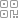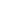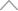6 0 7 1 4 1 4

• 1 引言

• 2 分类问题

• 3 回归问题

• 4 树剪枝

• 5 总结

2 分类问题

X

X

L

L

X

X

G i n i ( X ) = l L | X l | | X | ( 1 | X l | | X | ) = 1 l = 1 L ( | X l | | X | ) 2

G

i

n

i

(

X

)

=

l

L

|

X

l

|

|

X

|

(

1

|

X

l

|

|

X

|

)

=

1

l

=

1

L

(

|

X

l

|

|

X

|

)

2

A

A

X

X

a

a

A

A

X

X

a

a

X 1 = { x X | x A = a }

X

1

=

{

x

X

|

x

A

=

a

}

X 2 = { x X | x A a }

X

2

=

{

x

X

|

x

A

a

}

，那么在特征

A

A

X

X

G i n i ( X , A ) = X 1 X G i n i ( X 1 ) + X 2 X G i n i ( X 2 )

G

i

n

i

(

X

,

A

)

=

|

X

1

X

|

G

i

n

i

(

X

1

)

+

|

X

2

X

|

G

i

n

i

(

X

2

)A

A

G i n i ( X , A 1 ) = 5 10 × { 2 × 4 5 × 1 5 } + 5 10 × { 2 × 3 5 × 2 5 } = 0.4

G

i

n

i

(

X

,

A

1

)

=

5

10

×

{

2

×

4

5

×

1

5

}

+

5

10

×

{

2

×

3

5

×

2

5

}

=

0.4

A

A

G i n i ( X , A 2 ) = G i n i ( X , A 1 ) = 0.4

G

i

n

i

(

X

,

A

2

)

=

G

i

n

i

(

X

,

A

1

)

=

0.4

B

B

G i n i ( X , B 1 ) = 3 10 × { 2 × 2 3 × 1 3 } + 7 10 × { 2 × 5 7 × 2 7 } = 0.42

G

i

n

i

(

X

,

B

1

)

=

3

10

×

{

2

×

2

3

×

1

3

}

+

7

10

×

{

2

×

5

7

×

2

7

}

=

0.42

B

B

G i n i ( X , B 2 ) = 4 10 × { 2 × 4 4 × 0 4 } + 6 10 × { 2 × 3 6 × 3 6 } = 0.3

G

i

n

i

(

X

,

B

2

)

=

4

10

×

{

2

×

4

4

×

0

4

}

+

6

10

×

{

2

×

3

6

×

3

6

}

=

0.3

B

B

G i n i ( X , B 3 ) = 3 10 × { 2 × 1 3 × 2 3 } + 7 10 × { 2 × 6 7 × 1 7 } = 0.46

G

i

n

i

(

X

,

B

3

)

=

3

10

×

{

2

×

1

3

×

2

3

}

+

7

10

×

{

2

×

6

7

×

1

7

}

=

0.46

C

C

G i n i ( X , C 1 ) = 3 10 × { 2 × 0 3 × 3 3 } + 7 10 × { 2 × 4 7 × 3 7 } = 0.34

G

i

n

i

(

X

,

C

1

)

=

3

10

×

{

2

×

0

3

×

3

3

}

+

7

10

×

{

2

×

4

7

×

3

7

}

=

0.34

C

C

G i n i ( X , C 2 ) = 3 10 × { 2 × 1 3 × 2 3 } + 7 10 × { 2 × 5 7 × 2 7 } = 0.42

G

i

n

i

(

X

,

C

2

)

=

3

10

×

{

2

×

1

3

×

2

3

}

+

7

10

×

{

2

×

5

7

×

2

7

}

=

0.42

C

C

G i n i ( X , C 3 ) = 3 10 × { 2 × 1 3 × 2 3 } + 7 10 × { 2 × 6 7 × 1 7 } = 0.46

G

i

n

i

(

X

,

C

3

)

=

3

10

×

{

2

×

1

3

×

2

3

}

+

7

10

×

{

2

×

6

7

×

1

7

}

=

0.46

B

B

3 回归问题

x X

x

X

，经过决策树后的输出

f ( x )

f

(

x

)

f ( x )

f

(

x

)

X

X

f ( x )

f

(

x

)

f ( x )

f

(

x

)

X

X

，假设我们在其特征属性

A

A

a

a

X 1 = { x | x A a }

X

1

=

{

x

|

x

A

a

}

X 2 = { x | x A > a }

X

2

=

{

x

|

x

A

>

a

}

f ( x )

f

(

x

)

c 1

c

1

c 2

c

2

，那么根据特征属性

A

A

a

a

X

X

L o s s A , a = x X 1 ( y c 1 ) 2 + x X 2 ( y c 2 ) 2

L

o

s

s

A

,

a

=

x

X

1

(

y

c

1

)

2

+

x

X

2

(

y

c

2

)

2

y

y

x

x

L o s s A , a

L

o

s

s

A

,

a

c 1

c

1

c 2

c

2

，目标函数为：

min x X 1 ( y c 1 ) 2 + min x X 2 ( y c 2 ) 2

min

x

X

1

(

y

c

1

)

2

+

min

x

X

2

(

y

c

2

)

2

c 1

c

1

c 2

c

2

L o s s A , a

L

o

s

s

A

,

a

c 1

c

1

c 2

c

2

X 1

X

1

X 2

X

2

y

y

c 1

c

1

c 2

c

2

c i = a v e ( y | x X i )

c

i

=

a

v

e

(

y

|

x

X

i

)

a

a

y

y

f ( x ) = c i = a v e ( y | x X i )

f

(

x

)

=

c

i

=

a

v

e

(

y

|

x

X

i

)

X

X

A

A

，计算其所有取值

a

a

L o s s A , a

L

o

s

s

A

,

a

，然后对比所有

L o s s A , a

L

o

s

s

A

,

a

，取值最小的

L o s s A , a

L

o

s

s

A

,

a

A

A

a

a

（1）对当前数据集

X

X

，计算所有特征属性

A

A

a

a

L o s s A , a

L

o

s

s

A

,

a

（2）对比所有

L o s s A , a

L

o

s

s

A

,

a

，选择最小的

L o s s A , a

L

o

s

s

A

,

a

A

A

a

a

（3）对左右两个子树的数据集重复（1）、（2）步骤继续划分，直到节点中数据集满足指定条件则决策树构建完成。

4 树剪枝

T 0

T

0

T α

T

α

（1）令

k = 0

k

=

0

T = T 0

T

=

T

0

α = +

α

=

+

（2）自上而下地对各内部节点计算

C ( T t )

C

(

T

t

)

| T t |

|

T

t

|

g ( t ) = C ( t ) C ( T t ) | T t | 1

g

(

t

)

=

C

(

t

)

C

(

T

t

)

|

T

t

|

1

α = min ( α , g ( t ) )

α

=

min

(

α

,

g

(

t

)

)

T t

T

t

t

t

C ( t )

C

(

t

)

t

t

C ( T t )

C

(

T

t

)

| T t |

|

T

t

|

T t

T

t

（3）自上而下地访问内部节点

t

t

，如果有

g ( t ) = α

g

(

t

)

=

α

，则对

t

t

t

t

T

T

（4）令

k = k + 1

k

=

k

+

1

α k = α

α

k

=

α

T k = T

T

k

=

T

（5）如果

T

T

（6）采用交叉验证法在子树序列

T 0 , T 1 , , T k = T

T

0

,

T

1

,

,

T

k

=

T

T α

T

α

g ( t )

g

(

t

)

g ( t )

g

(

t

)

t

t

t

t

g ( t )

g

(

t

)

g ( t )

g

(

t

)

g ( t )

g

(

t

)

t

tα 1 = 0

α

1

=

0

，树中共有3个节点，对每个节点分别计算其

g ( t )

g

(

t

)t 1

t

1

t 2

t

2

g ( t )

g

(

t

)

t 3

t

3

α 2 = 1 8

α

2

=

1

8

。剪枝后决策树如下：g ( t )

g

(

t

)

:t 2

t

2

g ( t )

g

(

t

)

t 2

t

2

α 3 = 1 8

α

3

=

1

8t 1

t

1

，计算后有

g ( t 3 ) = 1 4

g

(

t

3

)

=

1

4

，所以

α 4 = 1 4

α

4

=

1

4

α 0 = 0 , α 2 = 1 8 , α 3 = 1 8 , α 4 = 1 4

α

0

=

0

,

α

2

=

1

8

,

α

3

=

1

8

,

α

4

=

1

4

，以及对应的子树。接下来剩余的工作就是利用独立的验证数据集计算每个子树的平方误差或者基尼指数，选择误差最小的那个子树作为最优的剪枝后的树。

5 总结新知精选

IT研发知识▪优秀创作者

优选课程 新知学院

•扫码下载商业新知APP~

•回到顶部